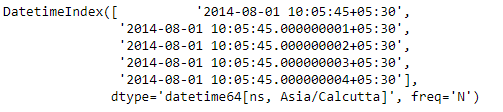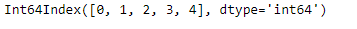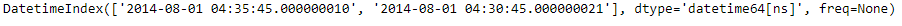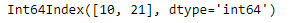Python | Pandas DatetimeIndex.nanosecond

• Last Updated : 24 Dec, 2018

Python is a great language for doing data analysis, primarily because of the fantastic ecosystem of data-centric python packages. Pandas is one of those packages and makes importing and analyzing data much easier.

Pandas DatetimeIndex.nanosecond attribute outputs an Index object containing the nanosecond values present in each of the entries of the DatetimeIndex object.

Syntax: DatetimeIndex.nanosecond

Return: Index containing nanosecond.

Example #1: Use DatetimeIndex.nanosecond attribute to find the nanosecond value present in the DatetimeIndex object.

 # importing pandas as pdimport pandas as pd  # Create the DatetimeIndex# Here 'N' represents nanosecondsdidx = pd.DatetimeIndex(start ='2014-08-01 10:05:45', freq ='N',                              periods = 5, tz ='Asia/Calcutta')  # Print the DatetimeIndexprint(didx)

Output :Now we want to find all the nanoseconds value present in the DatetimeIndex object.

 # find all the nanosecond values present in the objectdidx.nanosecond

Output :As we can see in the output, the function has returned an Index object containing the nanosecond values present in each entry of the DatetimeIndex object.

Example #2: Use DatetimeIndex.nanosecond attribute to find the nanosecond value present in the DatetimeIndex object.

 # importing pandas as pdimport pandas as pd  # Create the DatetimeIndexdidx = pd.DatetimeIndex(['2014-08-01 10:05:45.000000010 + 05:30',                         '2014-08-01 10:05:45.000000021 + 05:35'])  # Print the DatetimeIndexprint(didx)

Output :Now we want to find all the nanoseconds value present in the DatetimeIndex object.

 # find all the nanosecond values present in the objectdidx.nanosecond

Output :As we can see in the output, the function has returned an Index object containing the nanosecond values present in each entry of the DatetimeIndex object.

My Personal Notes arrow_drop_up